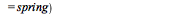New Features in Maple 2018 - GraphTheory - Maplesoft

GraphTheory

The Graph Theory package is a collection of routines for creating, drawing, and manipulating graphs, and for testing graphs for particular properties.  Maple 2018 enhances the GraphTheory package with new functions, including:

• CliquePolynomial
• DistancePolynomial
• FindClique
• GraphIntersection
• IndependencePolynomial
• IsReachable
• Reachable

The SpecialGraphs subpackage also includes support for seven new graphs and families of graphs.

Examples

FindClique

FindClique returns a list of vertices which comprise a clique in the graph G. The optional parameter size specifies a size for the clique.

 > with(GraphTheory):
> G := GraphComplement(CompleteGraph(3,4));> DrawGraph(G);> FindClique(G, 3);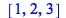> FindClique(G, 4);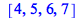GraphIntersection

GraphIntersection returns a graph G which is the intersection of the graphs G1,...,Gs, such that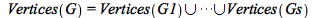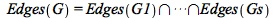> G1 := Graph(5,{{1,2},{1,3},{1,4},{1,5}});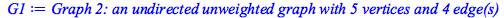> G2 := Graph(5,{{1,2},{1,3},{1,4},{1,5},{2,3},{3,4},{4,5},{5,2}});> DrawGraph(G1);> DrawGraph(G2);> DrawGraph(GraphIntersection(G1,G2));IndependencePolynomial

IndependencePolynomialreturns the independence polynomial for the graph G in the variable x.

 > with(SpecialGraphs):
 > P := Graph( {{1,2},{2,3},{3,4}} ); # a path> IndependencePolynomial(P,x);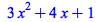&
 > C := CycleGraph( 5 ); # a cycle> IndependencePolynomial(C,x);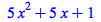New Special Graphs

The SpecialGraphs subpackage now includes built-in commands to generate the following special graphs or families of special graphs:

Doyle Graph

Gear Graph

Gray Graph

Nauru Graph

 >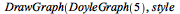>>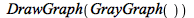>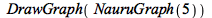Poussin Graph

Turan Graph

Tutte Graph

 >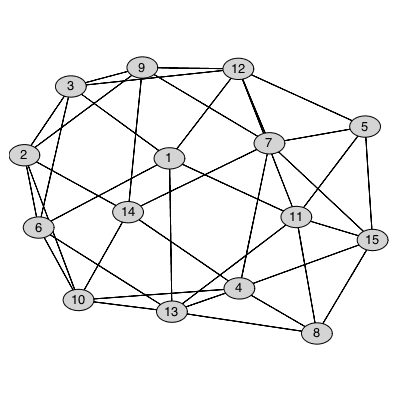>>